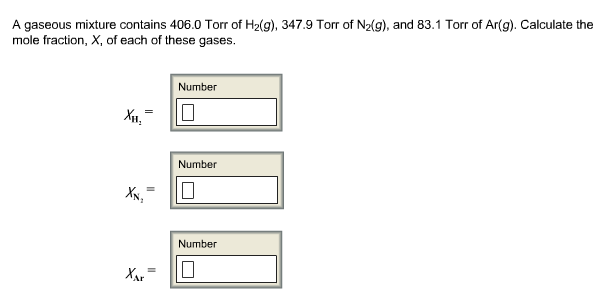# Problem: A gaseous mixture contains 406.0 Torr of H2(g), 347.9 Torr of N2 (g), and 83.1 Torr of Ar(g). Calculate the mole fraction, X, of each of these gases.XH2 =XN2 =XAr =

###### FREE Expert Solution
95% (477 ratings)###### Problem Details

A gaseous mixture contains 406.0 Torr of H2(g), 347.9 Torr of N2 (g), and 83.1 Torr of Ar(g). Calculate the mole fraction, X, of each of these gases.

XH2 =

XN2 =

XAr =